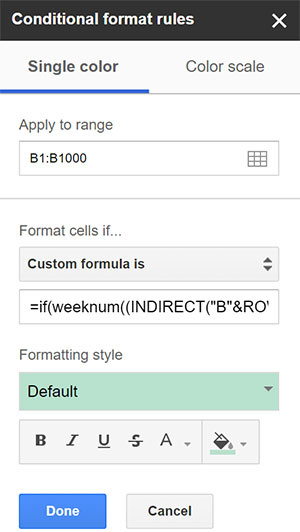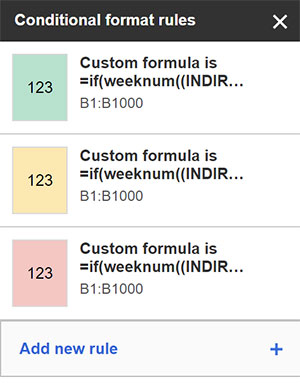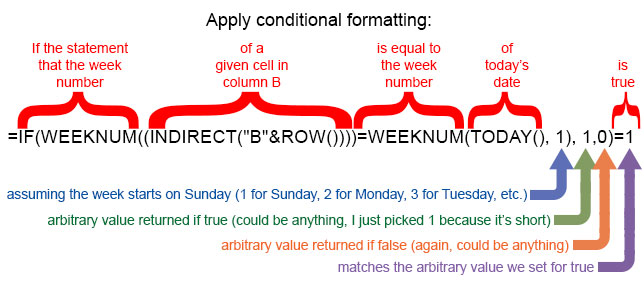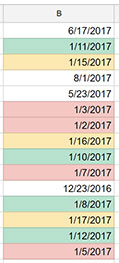Tutorial

### The Very Basics of Conditional Formatting

Conditional formatting is used when you want to apply styles to a large number of cells using data within cells to determine how a cell is formatted. Maybe you want to draw attention to dates that are getting near, such as a deadline, or maybe you want to color a range of cells based on a "high-priority" label or something similar.

Using conditional formatting is fairly simple for most applications. Just select a range of cells (including whole columns or groups of columns), then choose Format > Conditional formatting from the menu.

From there, you can use any of the pre-defined custom formatting options provided by Google, or you can write your own custom formulas for more complex interactions.

We've covered a lot about using custom formulas for conditional formatting in Google Sheets, especially color-coding cells based on the date. Now let's look at how to color-code cells based on whether a date contained in a cell falls in this week, next week, last week or any other week.

For this tutorial, we'll shade a cell green if the date in that cell is in this week, yellow if it's next week and red if it's last week. For this example, we'll put our date fields in column B and also color-code those date fields in column B. Note that the dates can be in any column you choose, and you can color code any column based on the date in the same or any other column.

So the basic formula we want to create will color a cell in column B if a date in column B falls in the same week as today's date.

### Long Story Short

For those of you who aren't interested in the whys or hows, here are the formulas you can plug into your Custom Formula field in the Conditional Formatting dialog.

For a date that occurs within the current week, assuming the week starts on a Sunday and ends on a Saturday:

=IF(WEEKNUM((INDIRECT("B"&ROW())))=WEEKNUM(TODAY(), 1), 1,0)=1

For a date that occurs next week:

=if(WEEKNUM((INDIRECT("B"&ROW())))=WEEKNUM((TODAY()+7), 1), 1,0)=1

For a date that occurred last week:

=if(WEEKNUM((INDIRECT("B"&ROW())))=WEEKNUM((TODAY()-7), 1), 1,0)=1Paste the formula into the Conditional
formatting pane as seen above.

### Long Story Long

For those who want to understand how this works so you can extrapolate other uses, here's what all of that means.

To make this work, we need a few operators:

• WEEKNUM, which we have not used before, determines which week of the year we are in now and which week the date in a specific cell falls in and is essential to this formula. IRL we are in week 2 at the time I am posting this (Jan. 12). Entering "=WEEKNUM(TODAY(), 1)" (without the quotes) in any cell in your spreadsheet should result in a number appearing in that cell representing the current week number of the current year, e.g. 2 if you are trying this in the second week of January.
• IF, which we've used in past tutorials, will determine if the statement is true of false that a date in column B is within the current week. IF essentially works like this: IF([a whole bunch of stuff], is true then x value, is false then Y value). Example: If you type "=IF(B1=C1, 1, 0)" in any cell in your spreadsheet other than B1 or C1, then either a 1 or a 0 will appear in your cell, depending on whether it's true or false that the value in cell B1 equals the value in cell C1.
•to the Conditional formatting pane.
Rules are evaluated in the order they
appear in the pane.

• INDIRECT lets us apply our formatting to any cell in a given column rather than specifying a cell number for each individual cell (we've used this one before). So if we want to expand the above formula to check a cell in B in any given row against a cell in C in the same row without specifying a row, we do this: "=IF((INDIRECT("B"&ROW()))=(INDIRECT("C"&ROW())), 1, 0)". Place that formula in D1, and it will check B1 against C1. Place it in D2 and it will check B2 against C2. In a custom formula for a conditional format, that requires one minor modification: "=IF((INDIRECT("B"&ROW()))=(INDIRECT("C"&ROW())), 1, 0)=1" (which essentially applies the conditional format only f the statement is true and doesn't apply it if it's false). Place that formula in the conditional formatting pane for the entire column D, and it will check every cell in B against its adjacent cell in C and apply formatting where appropriate (i.e. where the value in a cell in B is equal to the value in cell C in the same row).
• TODAY just calls up today's date (which, again, we've used in the past) and will be used to determine what current week we are in. Put "=TODAY()" in any cell, and today's date will appear. Place "=TODAY()+7" in a cell, and the date seven days from now will appear.

So, looking at the formula for this week, we can break it down as follows:So how would you extrapolate from that to compare a date to next week instead of this week?The formatted cells.

The portion "WEEKNUM(TODAY(), 1)" means the week number of today's date, given that the week starts on Sunday. So next week would simply be the date seven days later, which would be written: "WEEKNUM((TODAY()+7), 1)," with an extra set of parentheses around (TODAY()+7).

What about last week then? You guessed it: "WEEKNUM((TODAY()-7), 1)."

And you can extrapolate from there the method for formatting a date with any given week number.

You can find more tutorials on conditional formatting and the use of custom formulas on our Google G Suite tutorial archive.